Question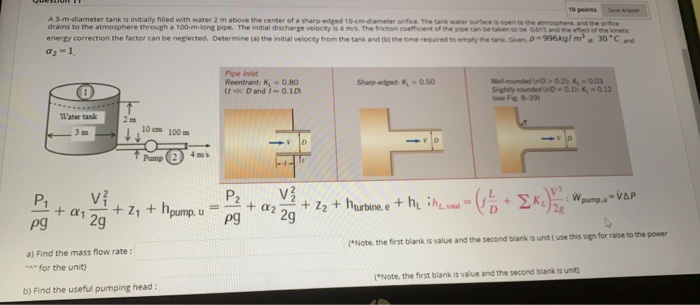We will take the point 1 at the free surface of the tank and point 2 at the exit of the pipe. after this centerline is taken at the centerline of the orific where Z2=0. Positive direction of z is taken upwards

Fluid velocity at free surface is very low and almost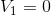Also the fluid at both the points is open to atmosphere so in that case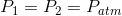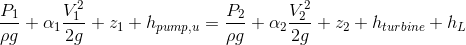After putting the above mentioned conditions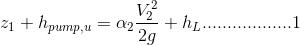where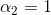Given

So from here the total head can be defined as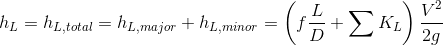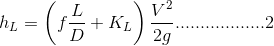Since the dia of the piping system is constant and the initial velocity is given as 4 m/s so from here required mass flow rate and head can be calculated as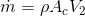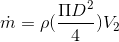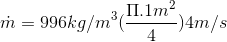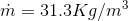Answer

Now from equation 1 and 2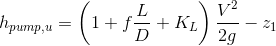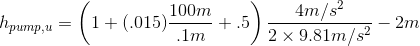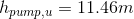Answer

#### Earn Coins

Coins can be redeemed for fabulous gifts.

Similar Homework Help Questions
• ### A 3-m-diameter tank is initially filled with water 2 m above the center of a sharp-edged 10-cm-diameter orifice. The tank water surface is open to the atmosphere, and the orifice drains to the at...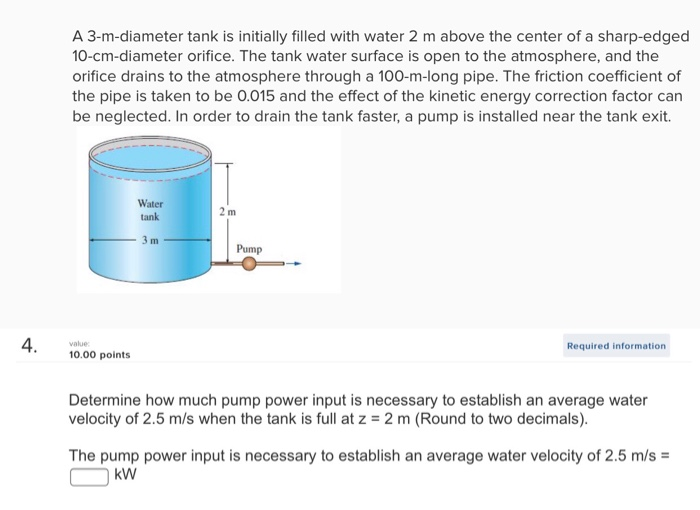A 3-m-diameter tank is initially filled with water 2 m above the center of a sharp-edged 10-cm-diameter orifice. The tank water surface is open to the atmosphere, and the orifice drains to the atmosphere through a 100-m-long pipe. The friction coefficient of the pipe is taken to be 0.015 and the effect of the kinetic energy correction factor can be neglected. In order to drain the tank faster, a pump is installed near the tank exit. Water tank Pump Required...

• ### A 3-m-diameter tank is initially filled with water 2 m above the center of a sharp-edged 10-cm-diameter orifice. The tank water surface is open to the atmosphere, and the orifice drains t...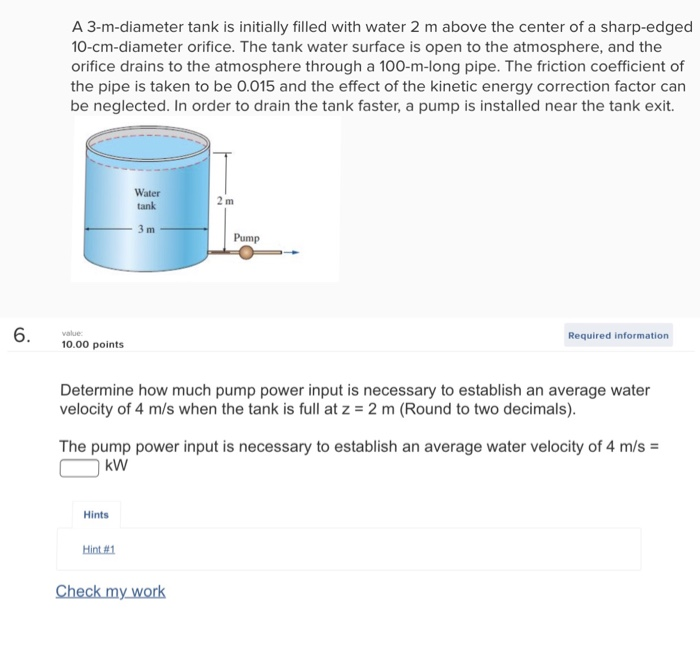A 3-m-diameter tank is initially filled with water 2 m above the center of a sharp-edged 10-cm-diameter orifice. The tank water surface is open to the atmosphere, and the orifice drains to the atmosphere through a 100-m-long pipe. The friction coefficient of the pipe is taken to be 0.015 and the effect of the kinetic energy correction factor can be neglected. In order to drain the tank faster, a pump is installed near the tank exit. Water tank Pump 6...

• ### A 3-m-diameter tank is initially filled with water 2 m above the center of a sharp-edg...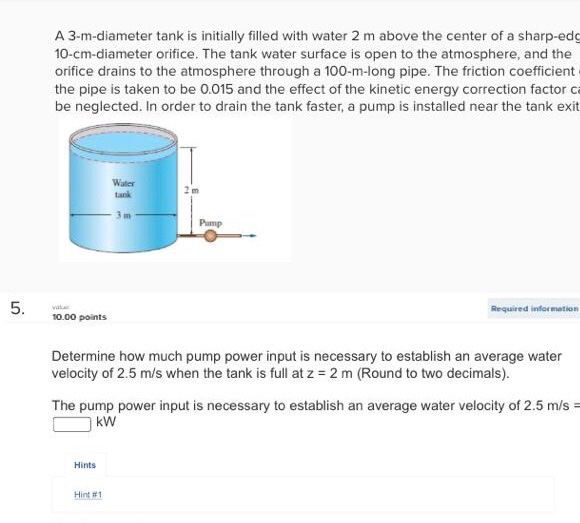A 3-m-diameter tank is initially filled with water 2 m above the center of a sharp-edg 10-cm-diameter orifice. The tank water surface is open to the atmosphere, and the orifice drains to the atmosphere through a 100-m-long pipe. The friction coefficient the pipe is taken to be 0.015 and the effect of the kinetic energy correction factor ca be neglected. In order to drain the tank faster, a pump is installed near the tank exit Water tank Pamp Required infor...

• ### 2. Find the time taken by water for completely emptying to tank. Take C 0.6 12 ft 10 ft Water 4-diameter, sharp-edg...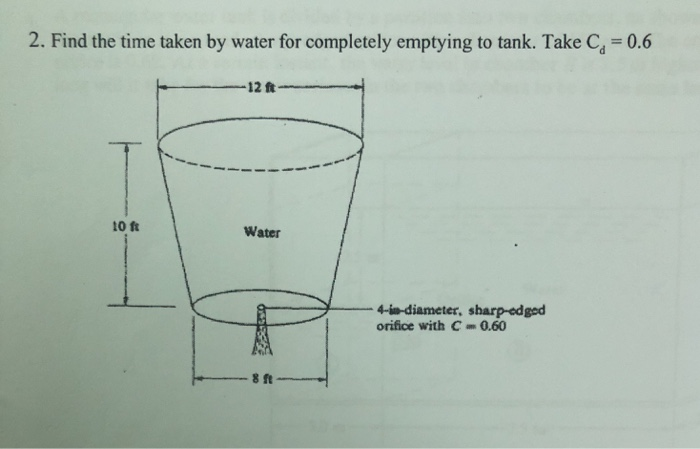2. Find the time taken by water for completely emptying to tank. Take C 0.6 12 ft 10 ft Water 4-diameter, sharp-edged orifice with C 0.60

• ### A water tank filled with solar-heated water at 40°C is to be used for showers in...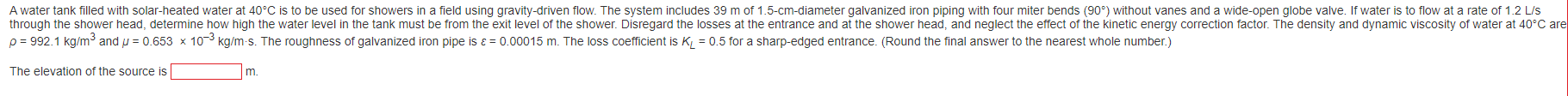A water tank filled with solar-heated water at 40°C is to be used for showers in a field using gravity-driven flow. The system includes 39 m of 1.5-cm-diameter galvanized iron piping with four miter bends (90°) without vanes and a wide-open globe valve. If water is to flow at a rate of 1.2 L/S through the shower head, determine how high the water level in the tank must be from the exit level of the shower. Disregard the losses at...

• ### Problem 4 (50 points) water is discharged from a reservoir at a rate of 18.103 m2/s using two horizontal cast iron pipes nected in series and a pump between them. The first pipe is 20 m long and...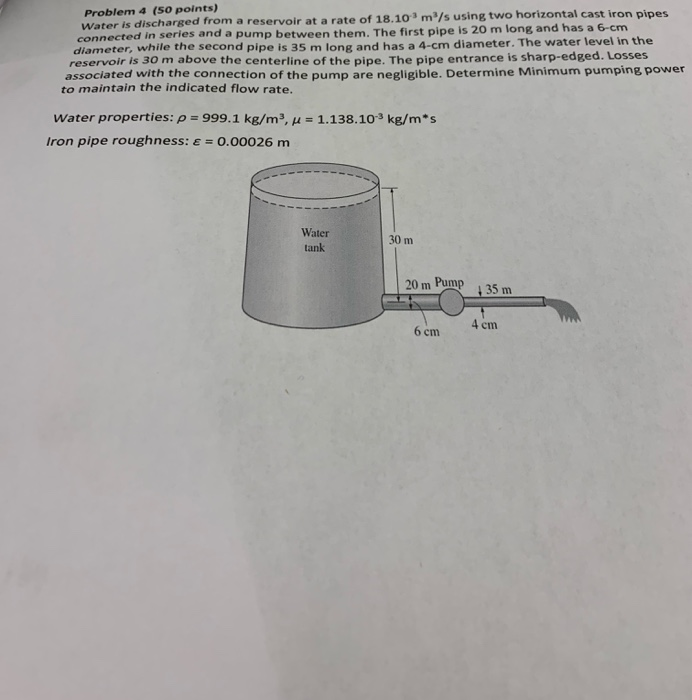Problem 4 (50 points) water is discharged from a reservoir at a rate of 18.103 m2/s using two horizontal cast iron pipes nected in series and a pump between them. The first pipe is 20 m long and has a 6-cm diameter, while the second pipe is 35 m long and has a 4-cm diameter. The water level in the reservoir is 30 m above the centerline of the pipe. The pipe entrance is sharp-edged. Losses associated with the connection...

• ### Two water reservoirs A and B are connected to each other through a 35m-long, 2-cm-diameter cast...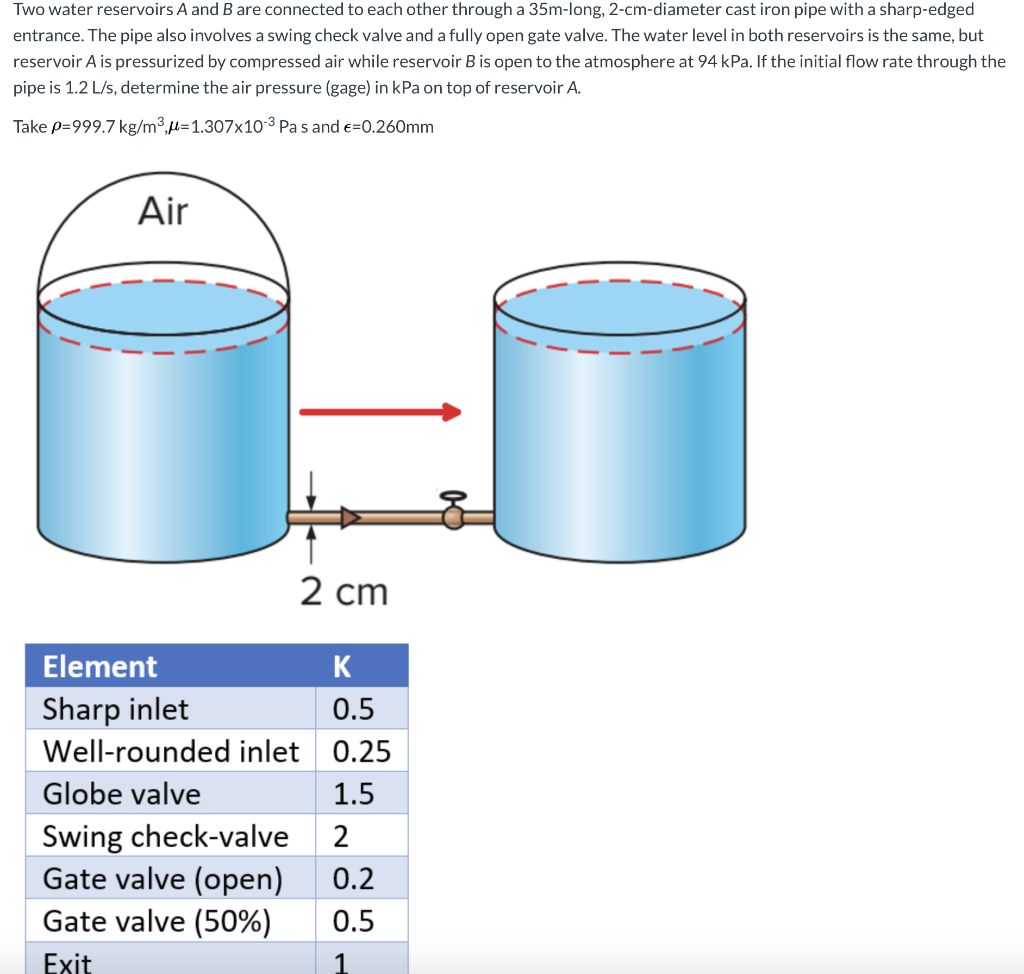Two water reservoirs A and B are connected to each other through a 35m-long, 2-cm-diameter cast iron pipe with a sharp-edged entrance. The pipe also involves a swing check valve and a fully open gate valve. The water level in both reservoirs is the same, but reservoir A is pressurized by compressed air while reservoir B is open to the atmosphere at 94 kPa. If the initial flow rate through the pipe is 1.2 L/s, determine the air pressure (gage)...

• ### Can I know full answer 2. Pressurized tank of water has a 10-cm-diameter orifice at the...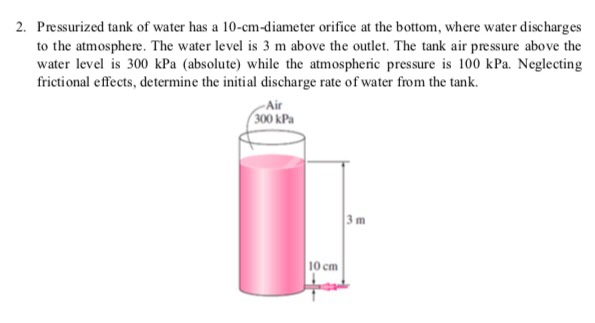Can I know full answer 2. Pressurized tank of water has a 10-cm-diameter orifice at the bottom, where water discharges to the atmosphere. The water level is 3 m above the outlet. The tank air pressure above the water level is 300 kPa (absolute) while the atmospheric pressure is 100 kPa. Neglecting frictional effects, determine the initial discharge rate of water from the tank. Air 00 kPa 3 m 10 cm

• ### 1. Atall cylindrical tank, held above ground on stilts, is partially filled with water. (See top...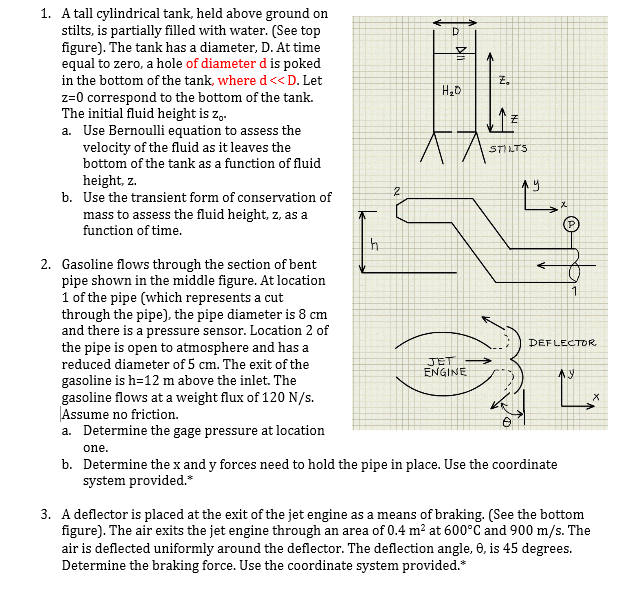1. Atall cylindrical tank, held above ground on stilts, is partially filled with water. (See top figure). The tank has a diameter, D. At time equal to zero, a hole of diameter d is poked in the bottom of the tank, where d<< D. Let z-0 correspond to the bottom of the tank. The initial fluid height is za. Had Use Bernoulli equation to assess the velocity of the fluid as it leaves the bottom of the tank as a...

• ### The total length of the piping system made of cast iron providing hot water to a...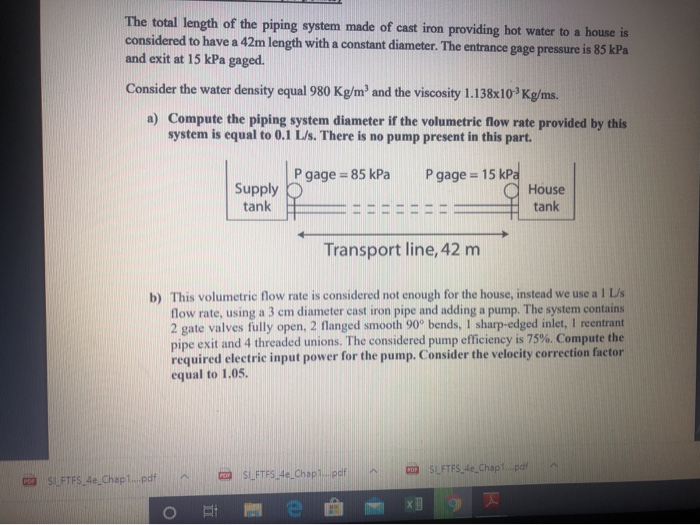The total length of the piping system made of cast iron providing hot water to a house is considered to have a 42m length with a constant diameter. The entrance gage pressure is 85 kPa and exit at 15 kPa gaged. Consider the water density equal 980 Kg/m' and the viscosity 1.138x10 Kg/ms. a) Compute the piping system diameter if the volumetric flow rate provided by this system is equal to 0.1 L/s. There is no pump present in this...